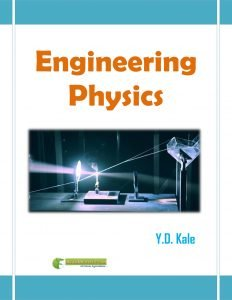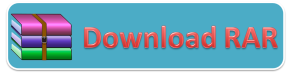You are here
Home > Book > ICAR eCourse > Agricultural Engineering > Engineering Physics (Agricultural Engineering) PDF Book Free Download

Book Detail: Engineering PhysicsLanguage: English

Pages: 143

Author: Y.D. Kale

Price: FreeOutlines of Engineering Physics

MODULE 1. Magnetism

LESSON 1. Basic concept of Magnetism & its terminology

LESSON 2. Classification of Magnetic Material

LESSON 3. Adiabatic Demagetization & its properties

LESSON 4. Theory of Magnetism

MODULE 2. Particle Physics

LESSON 5. Basic concept of Wave and Particle

LESSON 6. Theory of Wave and particle

LESSON 7. Heisenberg Uncertainty Principle

LESSON 8. Mathematical Derivation for Uncertainty Priciple

LESSON 9. Wave Function and its significance

LESSON 10. Derivation for Schrodinger Wave Equation

MODULE 3. Modern Physics

LESSON 11. Qualitative Explanation of Zeeman Effect

LESSON 12. Qualitative Explanation of Stark Effect

LESSON 13. Paschan Back Effect & Introduction to Raman Spectroscopy

LESSON 14. Practical view of Raman Spectroscopy

LESSON 15. Concept of Bloch Function & Bloch Electron

LESSON 16. Bands in Solid

MODULE 4. Semicoductor Physics

LESSON 17. Effective Mass and Mathematical Derivation

LESSON 18. Band Theory of Solid

LESSON 19. Classification of Solids & Type of Semiconductor

LESSON 20. Law of Mass Action

MODULE 5. Superconductivty

LESSON 21. Basic Concept of Superconductor

LESSON 22. Isotop Effect & Type of superconductor

LESSON 23. Application of Superconductor

LESSON 24. High Temperature SuperConductor (HTS)

MODULE 6. Optics

LESSON 25. Basic concept of LASER

LESSON 26. Types of LASER

LESSON 27. Application of LASER

LESSON 28. Introduction to Optical Fiber System

LESSON 29. Theory of Optical Fiber- Index Fiber

LESSON 30. Theory of Optical Fiber – Mode of Propagation

LESSON 31. Basic concept of Photometry

LESSON 32. Law of Photometry

Disclaimer:The information on this website does not warrant or assume any legal liability or responsibility for the accuracy, completeness or usefulness of the courseware contents.

The contents are provided free for noncommercial purpose such as teaching, training, research, extension and self learning.